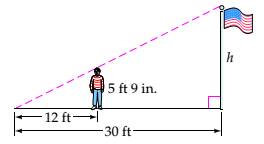Chapter 7.4, Problem 19ES### Mathematical Excursions (MindTap C...

4th Edition
Richard N. Aufmann + 3 others
ISBN: 9781305965584

#### Solutions

Chapter
Section### Mathematical Excursions (MindTap C...

4th Edition
Richard N. Aufmann + 3 others
ISBN: 9781305965584
Textbook Problem

# The given triangles are similar triangles. Use this fact to solve each exercise.Find the height of the flagpole.To determine

To find the height of the flagpole.

Explanation

Given information:

Given, the triangles are similar triangles.

And the given triangles are:

Calculation:

Labelling the vertices of the triangles:

When two triangles ABC and ADE are similar

Using the above rule:

Plugging the values,

1230=5

### Still sussing out bartleby?

Check out a sample textbook solution.

See a sample solution

#### The Solution to Your Study Problems

Bartleby provides explanations to thousands of textbook problems written by our experts, many with advanced degrees!

Get Started

## Additional Math Solutions

#### Find more solutions based on key concepts